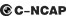5 种颜色可选2013款最低售价：7.79 万元起

4425(mm)1815(mm)1485(mm)##### 配置亮点：
• 胎压监测装置

• ISOFIX儿童座椅接口

• 车身稳定控制(ESC/ESP/DSC等)

• 电动天窗

• 定速巡航

• 后倒车雷达

• 真皮座椅

• GPS导航系统

• 氙气大灯

• 后视镜加热在售车型

排量 车型 厂商指导价 本地最低报价 购车工具
1.6L 1.6L 手动尚酷型 国IV 5挡手动 7.79万 7.79万

询底价+对比
1.6L 手动俊酷型 国IV 5挡手动 8.19万 8.19万

询底价+对比
1.6L 自动俊酷型 国IV 4挡手自一体 9.19万 9.19万

询底价+对比
1.6L 手动致酷型 国IV 5挡手动 8.79万 8.79万

询底价+对比
1.6L 自动致酷型 国IV 4挡手自一体 9.79万 9.79万

询底价+对比
1.6L 手动尚酷型 国V 5挡手动 7.89万 7.89万

询底价+对比
1.6L 手动俊酷型 国V 5挡手动 8.29万 8.29万

询底价+对比
1.6L 自动俊酷型 国V 4挡手自一体 9.29万 9.29万

询底价+对比
1.6L 手动致酷型 国V 5挡手动 8.89万 8.89万

询底价+对比
1.6L 自动致酷型 国V 4挡手自一体 9.89万 9.89万

询底价+对比
1.5T 1.5T 自动炫酷型 6挡手自一体 10.99万 10.99万

询底价+对比
1.5T 自动劲酷型 6挡手自一体 11.99万 11.99万

询底价+对比

经销商

查看更多 >>
更多 >>

本地降价排名

### 动力加速

0-100公里加速时间分布在 0.0-秒 属于 超跑级

动力级别 加速时间 车型

视频

新闻资讯

# 测试致尚XT 1.5T/1.6 MT 性能低于预期

评测 超过7463次关注

在我看来，致尚XT是自主品牌中为数不多的让人只看外形就十分心动的产品。不过人都是贪心的，尤其是看到自己心动的东西，所以我们直接向厂家借来了两辆致尚XT，准备...

# 长安致尚XT今晚上市 预计售价8.28万起

新闻 超过3400次关注

长安致尚XT今日正式上市，共7款车型，其中1.6L的五款，1.5T的两款，分别为1.6LMT尚酷型、1.6LMT俊酷型、1.6LMT致酷型、1.6LAT俊酷型、1.6LAT致酷型、1.5TAT炫酷型和...

# 长安致尚XT配置曝光 1.5T车型是亮点

新闻 超过3093次关注

近日，小编从经销商处获得了长安致尚XT的详细配置表。该车是逸动的两厢版车型，增加了1.5T+6AT动力组合，主打高性能运动风格，面向年轻群体。而从此次曝光的详细配...

# 长安致尚XT亮相上海 采用运动时尚设计

新闻 超过3443次关注

此次上海车展，长安携首款高性能两厢轿跑——致尚XT和我们见面。致尚XT虽然基于逸动平台打造，但为了与主攻家用的逸动区别开来，整车采用了更具运动感的掀背设计。

# 越短越美丽 试驾评测长安致尚XT两厢

试驾评测 超过3849次关注

我相信绝大部分见过致尚XT的朋友都不会掩饰对这款车的夸奖，事实的确如此，长安拿出了一款漂亮的两厢车，而且这一次他们还力推1.5T涡轮增压发动机，这俨然一款主打...

# 编辑心中最美两厢紧凑车型 长安致尚XT

导购 超过4226次关注

长安致尚XT全国优惠幅度：1千-5千元不等。

# C-NCAP最新碰撞解析 长安致尚XT解析

碰撞安全 超过4318次关注

致尚XT1.6AT致酷型碰撞成绩：53.3分5星。

猜你喜欢

﻿
• 快速找车
• 选择品牌
• 选择品牌
• A  奥迪
• A  阿斯顿·马丁
• A  阿尔法·罗密欧
• B  宝沃
• B  布加迪
• B  巴博斯
• B  保时捷
• B  宾利
• B  奔驰
• B  宝马
• B  本田
• B  别克
• B  标致
• B  比亚迪
• B  宝骏
• B  北汽制造
• B  北汽新能源
• B  北汽幻速
• B  北汽威旺
• B  北京汽车
• B  奔腾
• B  北汽绅宝
• C  长安
• C  长安商用
• C  长城
• C  昌河
• D  大众
• D  道奇
• D  DS
• D  东南
• D  东风风神
• D  东风风行
• D  东风小康
• D  东风风度
• D  东风
• F  福特
• F  丰田
• F  菲亚特
• F  法拉利
• F  福田
• F  福迪
• F  福汽启腾
• G  观致
• G  广汽传祺
• G  广汽吉奥
• G  GMC
• H  红旗
• H  汉腾汽车
• H  哈弗
• H  哈飞
• H  海格
• H  海马
• H  华颂
• H  黄海
• H  华泰
• H  恒天
• J  吉利汽车
• J  捷豹
• J  Jeep
• J  江淮
• J  江铃
• J  金杯
• J  九龙
• J  金旅
• K  凯翼
• K  凯迪拉克
• K  克莱斯勒
• K  科尼塞克
• K  卡威
• K  开瑞
• L  路虎
• L  林肯
• L  劳斯莱斯
• L  兰博基尼
• L  雷克萨斯
• L  铃木
• L  雷诺
• L  理念
• L  力帆
• L  莲花汽车
• L  猎豹
• L  路特斯
• L  陆风
• M  马自达
• M  MG
• M  MINI
• M  玛莎拉蒂
• M  摩根
• M  迈凯轮
• N  纳智捷
• O  欧宝
• O  讴歌
• O  欧朗
• Q  奇瑞
• Q  起亚
• Q  启辰
• R  日产
• R  荣威
• R  瑞麒
• S  三菱
• S  斯威汽车
• S  萨博
• S  smart
• S  斯柯达
• S  斯巴鲁
• S  思铭
• S  双龙
• S  上汽大通
• S  双环
• T  特斯拉
• T  腾势
• W  沃尔沃
• W  五菱汽车
• W  五十铃
• W  威兹曼
• W  威麟
• X  现代
• X  雪佛兰
• X  雪铁龙
• X  西雅特
• Y  一汽
• Y  英菲尼迪
• Y  英致
• Y  依维柯
• Y  野马汽车
• Y  永源
• Z  众泰
• Z  中华
• Z  中兴
• Z  知豆
• 选择车系
• 选择车系
• 车型对比
• 选择品牌
• 选择品牌
• A  奥迪
• A  阿斯顿·马丁
• A  阿尔法·罗密欧
• B  宝沃
• B  布加迪
• B  巴博斯
• B  保时捷
• B  宾利
• B  奔驰
• B  宝马
• B  本田
• B  别克
• B  标致
• B  比亚迪
• B  宝骏
• B  北汽制造
• B  北汽新能源
• B  北汽幻速
• B  北汽威旺
• B  北京汽车
• B  奔腾
• B  北汽绅宝
• C  长安
• C  长安商用
• C  长城
• C  昌河
• D  大众
• D  道奇
• D  DS
• D  东南
• D  东风风神
• D  东风风行
• D  东风小康
• D  东风风度
• D  东风
• F  福特
• F  丰田
• F  菲亚特
• F  法拉利
• F  福田
• F  福迪
• F  福汽启腾
• G  观致
• G  广汽传祺
• G  广汽吉奥
• G  GMC
• H  红旗
• H  汉腾汽车
• H  哈弗
• H  哈飞
• H  海格
• H  海马
• H  华颂
• H  黄海
• H  华泰
• H  恒天
• J  吉利汽车
• J  捷豹
• J  Jeep
• J  江淮
• J  江铃
• J  金杯
• J  九龙
• J  金旅
• K  凯翼
• K  凯迪拉克
• K  克莱斯勒
• K  科尼塞克
• K  卡威
• K  开瑞
• L  路虎
• L  林肯
• L  劳斯莱斯
• L  兰博基尼
• L  雷克萨斯
• L  铃木
• L  雷诺
• L  理念
• L  力帆
• L  莲花汽车
• L  猎豹
• L  路特斯
• L  陆风
• M  马自达
• M  MG
• M  MINI
• M  玛莎拉蒂
• M  摩根
• M  迈凯轮
• N  纳智捷
• O  欧宝
• O  讴歌
• O  欧朗
• Q  奇瑞
• Q  起亚
• Q  启辰
• R  日产
• R  荣威
• R  瑞麒
• S  三菱
• S  斯威汽车
• S  萨博
• S  smart
• S  斯柯达
• S  斯巴鲁
• S  思铭
• S  双龙
• S  上汽大通
• S  双环
• T  特斯拉
• T  腾势
• W  沃尔沃
• W  五菱汽车
• W  五十铃
• W  威兹曼
• W  威麟
• X  现代
• X  雪佛兰
• X  雪铁龙
• X  西雅特
• Y  一汽
• Y  英菲尼迪
• Y  英致
• Y  依维柯
• Y  野马汽车
• Y  永源
• Z  众泰
• Z  中华
• Z  中兴
• Z  知豆
• 选择车系
• 选择车系
• 选择车型
• 选择车型
• 意见反馈